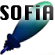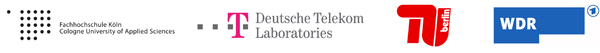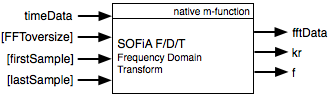Welcome to SOFiA
Who is behind SOFiA
Feature overview
System overview
Function reference
mergeArrayData
F/D/T
gauss
lebedev
S/W/G
S/T/C
W/G/C
S/F/E
M/F
R/F/I
P/D/C
I/T/C
makeMTX
makeIR
visual3D
Coordinate System
Application Examples
Example 1
Example 2
Example 3
Example 4
Example 5
Example 6
Example 7
Example 8
Array Datasets
VariSphear system
Groups and Mailinglists
Contact and Support
How to Reference

# SOFiA frequency domain transform

F/D/T transforms the time domain array data into spectral frequency domain data using the Fast Fourier Transform (FFT). As a default setting the full length impulse responses are tranformed with a Blocksize `NNFT=2^(nextpow2(lengthofimpulseresponses))`. The blocksize can be rised by using FFToversize which is a multiplier of NFFT (Default = 2). A limited time section can be picked out by defining `firstSample` and `lastSample`. The length of this section is `(lastSample-firstSample)`. This way unnecessary data can be removed or a running window can be realized to obtain time slices and resolve the temporal information within the captured sound field.

To save processing power and calculation time the SOFiA chain works on the half-sided FFT spectrum only (NFFT/2+1). Therefore F/D/T produces half-sided spectrum output signals (fftData). Later the makeIR function automatically reconstructs the double-sided spectrum to compute impulse responses.### ARGUMENTS

Input

Name Type Purpose Default
FFToversize int Rises the FFT Blocksize 2
firstSample int Start sample in time domain data 1
lastSample int End sample in time domain data length of timeData.ImpulseResponses

The structs contain the following fields:

 Field Type Purpose .impulseResponses float mtx Impulse Response Data N:Channels, M:Samples .FS int Sampling Frequency .downSample int Downsampling Factor (from Input) .averageAirTemp float Average air temperature .irOverlay float vector Overlay of all impulse responses (PLOT)

Output

Name Type Purpose
fftData complex float mtx Frequency domain data for S/T/C
kr float vec kr Values (k: waver number, r: radius)
f float vec Absolute frequency scale

## FILE(S):

 File Type OS/Matlab sofia_fdt.m Help header, Fuction All OS

 ```/* [ffftData, kr, f] = sofia_fdtVSA(timeData, FFToversize,...``` ``` ````firstSample, lastSample)` `------------------------------------------------------------------------------------` `fftData` `Frequency domain data ready for the SpatialFourier Transform (SOFiA S/T/C Core)!To save processing power the SOFiA chain alwaysworks on the half-sided FFT spectrum only (NFFT/2+1)` `kr` `kr-Values of the delivered data (required e.g. for the modal radial filters SOFiA M/F)` `f` `Absolute frequency scale. Not really required but good for control purposes or scaling a spectrum plot.` `------------------------------------------------------------------------------------` `timeData` ```Struct with minimum fields * . impulseResponses       [Channel X Samples]``` ``` FFTOversize``` ```FFToversize rises the FFT Blocksize. [default = 2] A FFT of the blocksize (FFToversize*NFFT) is applied to the time domain data; where NFFT is determinded as the next power of two of the signalSize which is signalSize = (lastSample-firstSample). The function will pick a window of (lastSample-firstSample) for the FFT: ``` ``` firstSamplelastSample``` ```First time domain sample to be included. Last time domain sample to be included. If firstSample and lastSample are not defined the full IR will be transformed: [default: firstSample = 1; lastSample = size(timeData.impulseResponses, 2);] ``` ``` Call this function with a running window (firstSample->lastSample+td) increasing td to obtain time slices. This way you resolve the temporal information within the captured sound field``` `*/`